## How to Calculate and Solve for Magnetic Flux Density or Field Induction | The Calculator Encyclopedia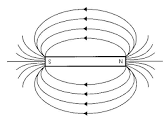The image above represents the magnetic flux density/field induction.

To compute the magnetic force of a field, four essential parameters are needed and the parameters are Magnetic Force (F), Quantity of Charge (q), Average Velocity of the Charge (v) and Angle between v and B (θ).

The formula for calculating the magnetic flux density/field induction:

B = F / qVsinθ

Where;
B = Magnetic Induction or Magnetic Flux Density
F = Magnetic Force
q = Quantity of Charge
v = Average Velocity of the charge
θ = Angle between v and B

Let’s solve an example;
Find the magnetic flux density/field induction of a field when Magnetic Force (F) is 17, Quantity of Charge (q) is 21, Average Velocity of the Charge (v) is 15 and Angle between v and B (θ) is 120°.

This implies that;
F = Magnetic Force = 17
q = Quantity of Charge = 21
v = Average Velocity of the charge = 15
θ = Angle between v and B = 120°

B = F / qVsinθ
B = 17 / (21 x 15)(sin 120°)
B = 17 / (315)(0.866)
B = 17 / (272.79)
B = 0.0623

Therefore, the magnetic flux density/field induction is 0.0623 Tesla.

## How to Calculate and Solve for the Current, Time and Quantity of Charge | Nickzom Calculator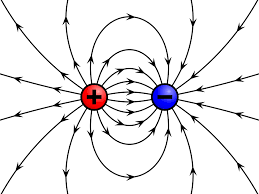The image above represents the quantity of charge.

To compute the quantity of charge, two essential parameters are needed and the parameters are current (I) and time (t).

The formula for calculating the quantity of charge;

Q = It

Where;
Q = Quantity of Charge
I = Current
t = Time

Let’s solve an example;
Find the quantity of charge when the current (I) is 24 amp with a time of 8 secs.

This implies that;
I = Current = 24 amp
t = Time = 8 secs

Q = It
Q = 24 x 8
Q = 192

Therefore, the quantity of charge is 192 coulombs (C).

Calculating the Current (I) of a charge using the Quantity of Charge (Q) and the time (t).

I = Q / t

Where;
I = Current
Q = Quantity of the charge
t = Time

Let’s solve an example;
Find the current of a charge with the quantity of the charge as 150 coulombs (C) and time as 15 secs.

This implies that;
Q = Quantity of the charge = 150 C
t = Time = 15 secs

I = Q / t
I = 150 / 15
I = 10

Therefore. the current is 10 Ampere (A).

## How to Calculate and Solve for Magnetic Force | Nickzom Calculator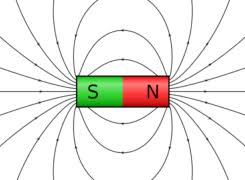The image above represents magnetic force.

To compute the magnetic force of a field, four essential parameters are needed and the parameters are Quantity of Charge (q), Average Velocity of the Charge (v), Magnetic Field Induction or Magnetic Flux Density (B) and Angle between v and B (θ).

The formula for calculating the magnetic force:

F = qVBsinθ

Where;
F = Magnetic Force
q = Quantity of Charge
v = Average Velocity of the Charge
B = Magnetic Field Induction or Magnetic Flux Density
θ = Angle between v and B

Let’s solve an example;
Find the magnetic force of a field when the Quantity of Charge (q) is 11, Average Velocity of the Charge (v) is 20, Magnetic Field Induction or Magnetic Flux Density (B) is 17 and Angle between v and B (θ) is 28°.

This implies that;
q = Quantity of Charge = 11
v = Average Velocity of the Charge = 20
B = Magnetic Field Induction or Magnetic Flux Density = 17
θ = Angle between v and B = 28°

F = qVBsinθ
F = 11 x 20 x 17 x sin28°
F = 11 x 20 x 17 x 0.469
F = 1755.82

Therefore, the magnetic force is 1755.82 Newton (N).

## How to Calculate and Solve for Escape Velocity | The Calculator Encyclopedia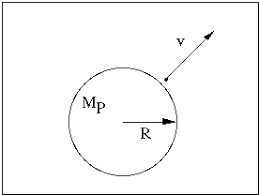The image above represents the escape velocity.

To compute the escape velocity of a field, two essential parameters are needed and the parameters are acceleration due to gravity (g) and radius (r).

The formula for calculating the escape velocity:

V = √(2gR)

Where;
V = Escape velocity
g = Acceleration due to gravity

Let’s solve an example;
Find the escape velocity of a field when the acceleration due to gravity is 12 and the radius is 24 cm.

This implies that;
g = Acceleration due to gravity = 12
r = Radius = 24 cm

V = √(2gR)
V = √(2 x 12 x 24)
V = √(576)
V = 24

Therefore, the escape velocity is 24 m/s.

## How to Calculate and Solve for Gravitational Potential | The Calculator Encyclopedia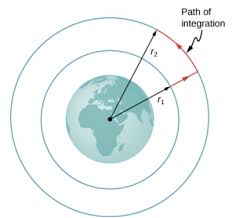The image above represents the Gravitational potential.

To compute the gravitational potential of a field, two essential parameters are needed which are mass (m) and radius (r).

The formula for calculating the gravitational potential;

V = (Gm) / r

Where;
V = Gravitational potential
m = Mass

Let’s solve an example;
Find the gravitational potential of a field when the mass is 14 cm with a radius of 9 cm.

This implies that;
m = Mass = 14 cm
r = Radius = 9 cm

V = (Gm) / r
V = (6.67 x 10-11 x 14) / 9
V = 1.0375e-10 / 9
V = 1.0375e-10

Therefore, the gravitational potential is 1.0375e-10 Volts (V).

## How to Calculate and Solve for Gravitational Force | The Calculator Encyclopedia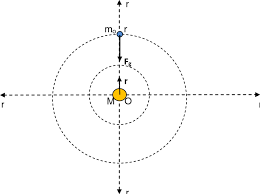The image above represents the gravitational force.

To compute the gravitational force of a field, three parameters are needed and this parameters are mass (m1), mass (m2) and radius between the masses (R).

The formula for calculating the gravitational force:

F = Gm1m2 /

Where;
F = Gravitational force
m1 = Mass 1
m2 = Mass 2
r = Radius between the masses

Let’s solve an example;
Find the gravitational force of a field when the mass 1 is 8 cm, mass 2 is 10 cm and the radius between masses is 14 cm.

This implies that;
m1 = Mass 1 = 8 cm
m2 = Mass 2 = 10 cm
r = Radius between the masses = 14 cm

F = Gm1m2 /
F = (6.67 x 10-11 x 8 x 10) / 196
F = 5.336e-9 / 196
F = 2.722e-11

Therefore, the gravitational force is 2.722e-11 Newton (N).

## How to Calculate and Solve for the Area of an Ellipsoid | The Calculator Encyclopedia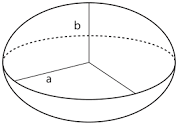The image above is an ellipsoid.

To compute the area of an ellipsoid, three essential parameters are needed and this parameters are axis (a)axis (b) and axis (c).

The formula for calculating the area of an ellipsoid:

A = 4π((ab)1.6 + (ac)1.6 + (bc)1.63)1 / 1.6

Where;
A = Area of the ellipsoid
a = Axis of the ellipsoid
b = Axis of the ellipsoid
c = Axis of the ellipsoid

Let’s solve an example;
Find the the area of an ellipsoid when the axis (a) of the ellipsoid is 12 cm, axis (b) of the ellipsoid is 6 cm and axis (c) of the ellipsoid is 2 cm.

This implies that;
a = Axis of the ellipsoid = 12 cm
b = Axis of the ellipsoid = 6 cm
c = Axis of the ellipsoid = 2 cm

A = 4π((ab)1.6 + (ac)1.6 + (bc)1.63)1 / 1.6
A = 4π(((12)(6))1.6 + ((12)(2))1.6 + ((6)(2))1.63)1 / 1.6
A = 4π(((72)1.6 + (24)1.6 + (12)1.6)3)1 / 1.6
A = 4π(((936.98) + (161.56) + (53.29))3)1 / 1.6
A = 4π((1151.84)3)1 / 1.6
A = 4π(383.946)1 / 1.6
A = 4π(383.946)0.625
A = 4π(41.2258)
A = (12.566)(41.2258)
A = 518.059

Therefore, the area of the ellipsoid is 518.059 cm2.

## How to Calculate and Solve for the Axis and Volume of an Ellipsoid | The Calculator EncyclopediaThe image above is an ellipsoid.

To compute the volume of an ellipsoid, three essential parameters are needed and this parameters are axis (a)axis (b) and axis (c).

The formula for calculating the volume of an ellipsoid:

V = 4πabc3

Where;

V = Volume of the ellipsoid
a = Axis of the ellipsoid
b = Axis of the ellipsoid
c = Axis of the ellipsoid

Let’s solve an example;
Given that the axis of the ellipsoid (a) is 9 cm and axis of the ellipsoid (b) is 11 cm with the axis (c) of 14 cm. Find the volume of the ellipsoid?

This implies that;
a = Axis of the ellipsoid = 9 cm
b = Axis of the ellipsoid = 11 cm
c = Axis of the ellipsoid = 14 cm

V = 4πabc3
V = 4π(9 x 11 x 14)3
V = 4π(1386)3
V = (12.56)(1386) / 3
V = (17416.98967) / 3
V = 5805.66

Therefore, the volume of the ellipsoid is 5805.66 cm3.

Calculating the Axis (a) of an Ellipsoid using the Volume of the Ellipsoid, Axis (b) of the Ellipsoid and Axis (c) of the Ellipsoid.

a = V3 / 4πbc

Where;
a = Axis of the ellipsoid
V = Volume of the ellipsoid
b = Axis of the ellipsoid
c = Axis of the ellipsoid

Let’s solve an example;
Find the axis (a) of an ellipsoid when the volume of the ellipsoid is 280 cm3 with an axis (b) of 18 cm and axis (c) of 8 cm.

This implies that;
V = Volume of the ellipsoid = 280 cm3
b = Axis of the ellipsoid = 18 cm
c = Axis of the ellipsoid = 8 cm

a = V3 / 4πbc
a = 280 x 3 / (12.566)(18 x 8)
a = 840 / (12.566)(144)
a = 840 / 1809.504
a = 0.46

Therefore, the axis (a) of the ellipsoid is 0.46 cm.